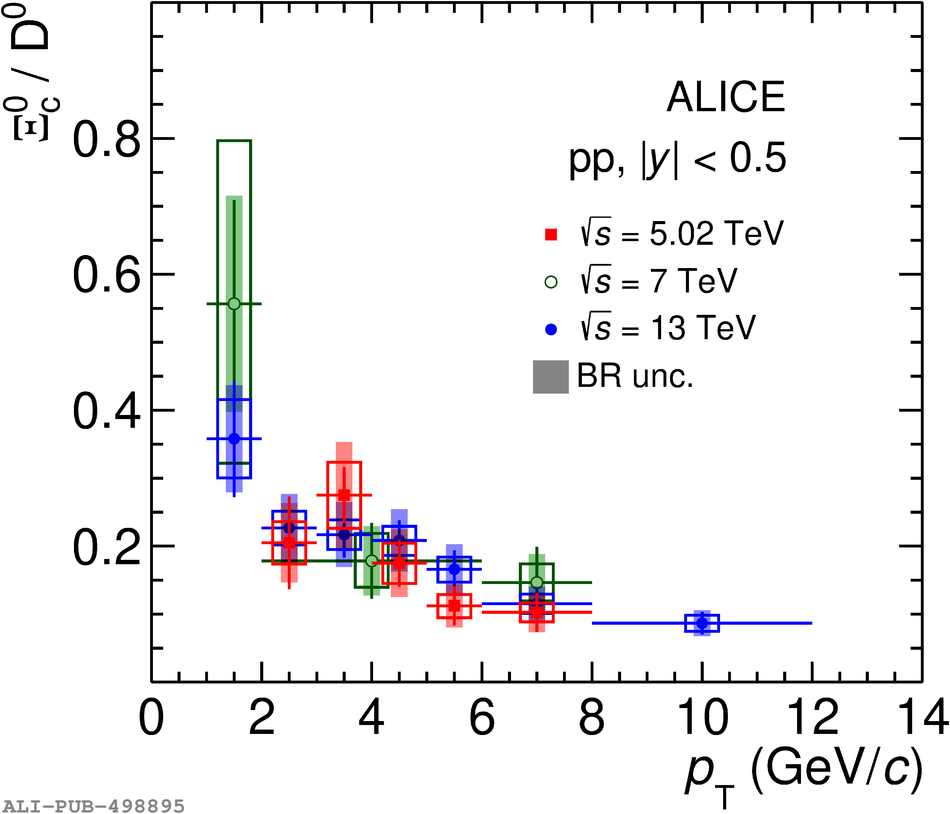# Figure 5

 Left panel: \pt-differential production cross sections of prompt $\Xi^0_{\rm c}$ baryons in pp collisions at $\sqrt{s}$ = 5.02 TeV and 13 TeV  and of inclusive $\Xi^0_{\rm c}$ baryons in pp collisions at $\sqrt{s}$ = 7 TeV   with updated decay BR as discussed in the text. The uncertainty of the BR of the cross sections of prompt $\Xi^0_{\rm c}$ baryons in pp collisions at $\sqrt{s}$ = 13 TeV is lower because it consists in the combination of two different decay channels ($\Xi^0_{\rm c} \rightarrow {\rm e}^{+}\Xi^{-}\nu_{\rm e}$ and $\Xi^0_{\rm c} \rightarrow {\pi}^{+}\Xi^{-}$)  Right panel: $\Xi^0_{\rm c}$/${\rm D^0}$ ratio measured in pp collisions at $\sqrt{s}$ = 5.02 TeV, compared with the measurements at $\sqrt{s}$ = 7 TeV  and $\sqrt{s}$ = 13 TeV . The uncertainty of the BR of ${\rm D^0}$ and $\Xi^0_{\rm c}$ are shown as shaded boxes.